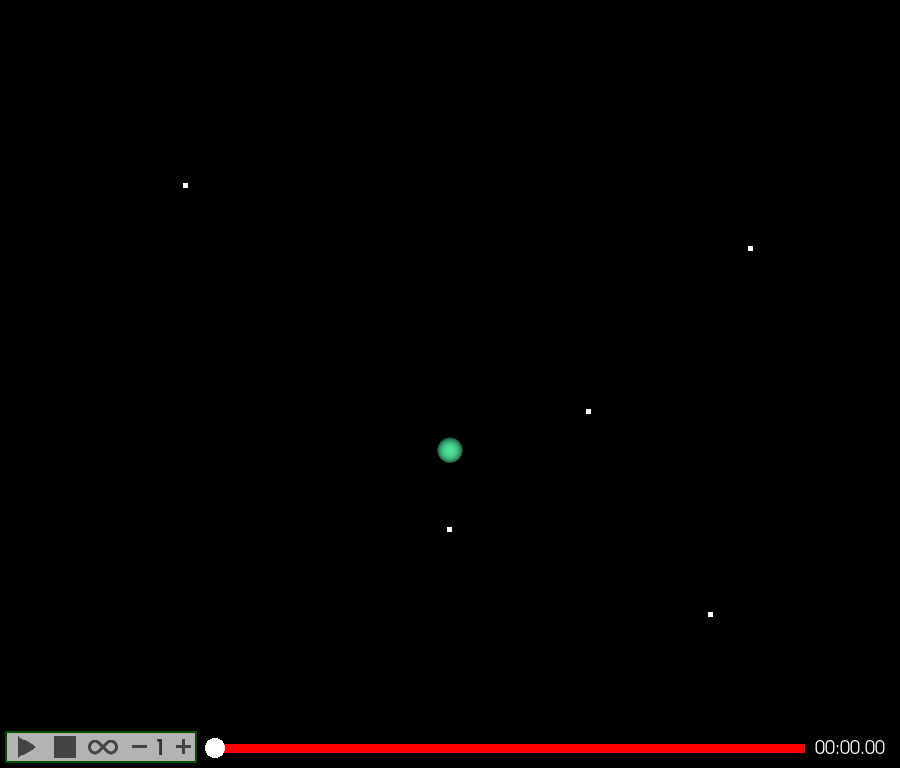# Keyframes Spline Interpolator#

Tutorial on making keyframe-based animation in FURY using Spline interpolators.

```import numpy as np
from fury import actor, window
from fury.animation import Animation, Timeline
from fury.animation.interpolator import spline_interpolator

scene = window.Scene()

showm = window.ShowManager(scene,
size=(900, 768), reset_camera=False,
order_transparent=True)
```

Position keyframes as a dict object containing timestamps as keys and positions as values.

```position_keyframes = {
0.0: np.array([0, 0, 0]),
2.0: np.array([10, 3, 5]),
4.0: np.array([20, 14, 13]),
6.0: np.array([-20, 20, 0]),
8.0: np.array([17, -10, 15]),
10.0: np.array([0, -6, 0]),
}
```

creating FURY dots to visualize the position values.

```pos_dots = actor.dot(np.array(list(position_keyframes.values())))
```

creating two timelines (one uses linear and the other uses’ spline interpolator), each timeline controls a sphere actor

```sphere_linear = actor.sphere(np.array([[0, 0, 0]]), (1, 0.5, 0.2), 0.5)

linear_anim = Animation()

linear_anim.set_position_keyframes(position_keyframes)
```

Note: linear_interpolator is used by default. So, no need to set it for this first animation that we need to linearly interpolate positional animation.

creating a second timeline that translates another larger sphere actor using spline interpolator.

```sphere_spline = actor.sphere(np.array([[0, 0, 0]]), (0.3, 0.9, 0.6), 1)
spline_anim = Animation(sphere_spline)
spline_anim.set_position_keyframes(position_keyframes)
```

Setting 5th degree spline interpolator for position keyframes.

```spline_anim.set_position_interpolator(spline_interpolator, degree=5)
```

## Wrapping animations up!#

Adding everything to a `Timeline` to control the two timelines.

First we create a timeline with a playback panel:

```timeline = Timeline(playback_panel=True)
```

Add visualization dots actor to the scene.

```scene.add(pos_dots)
```

Adding the animations to the timeline (so that it controls their playback).

```timeline.add_animation([linear_anim, spline_anim])
```

Adding the timeline to the show manager.

```showm.add_animation(timeline)
```

Now that these two animations are added to timeline, if the timeline is played, paused, …, all these changes will reflect on the animations.

```interactive = False

if interactive:
showm.start()

window.record(scene, out_path='viz_keyframe_animation_spline.png',
size=(900, 768))
```Total running time of the script: ( 0 minutes 0.090 seconds)

Gallery generated by Sphinx-Gallery